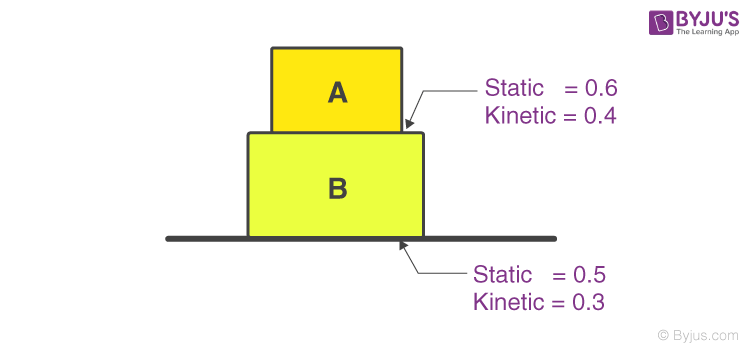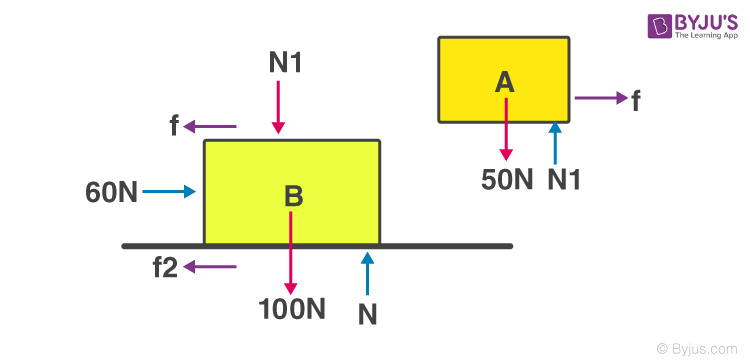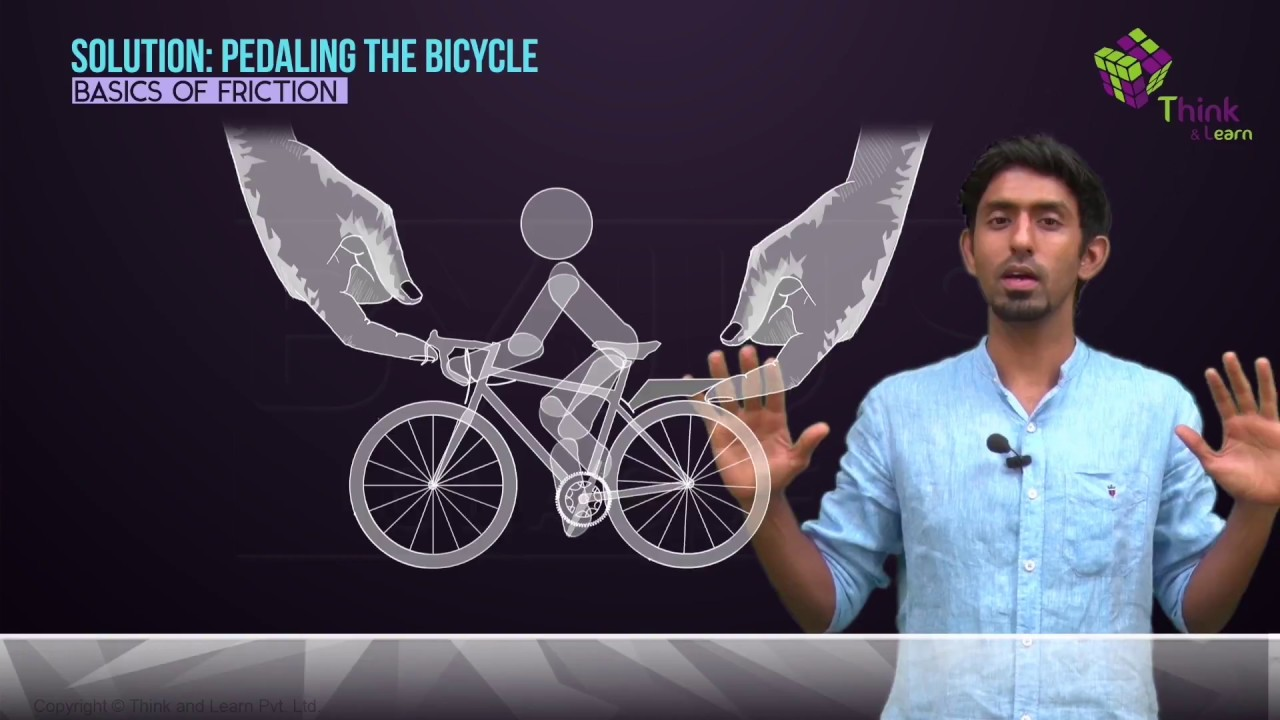# Friction

When we throw a ball on the floor, it starts moving with some velocity. But ideally, no force should be acting in the direction of motion, and according to Newton’s first law, the ball should keep rolling, but this does not happen. Instead, the ball stops after moving a certain distance, so a force must be acting on it. We call this force “friction.”

## What Is Friction?

Friction is defined as the resistance offered by the surfaces that are in contact when they move past each other.

Friction provides traction that is needed to walk without slipping. Friction is helpful in most cases. However, they also offer a great measure of opposition to the motion. In addition, about 20 per cent of the engine power of automobiles is consumed in overcoming frictional forces in the moving parts. In the next section, let us go through some of these factors.

## Factors Affecting Friction

Friction is a force that is dependent on external factors. Following are the two factors on which friction depends:

 1. On the nature of the two surfaces that are in contact Friction is dependent on the smoothness or roughness of the two surfaces that are in contact with each other. When the surface is smooth, the friction between the two reduces as there is not much interlocking of irregularities. While the surface is rough, friction increases. 2. On the force that is acting on these surfaces Friction increases when the force is applied along with the irregularities.

### What Causes Friction?

Friction is caused due to the irregularities on the two surfaces in contact. So, when one object moves over the other, these irregularities on the surface get entangled, giving rise to friction. The more the roughness, the more irregularities and more significant will be the friction.

## Types of Friction

There are four types of friction and they are classified as follows:

• Static friction
• Sliding friction
• Rolling friction
• Fluid friction

All four types of frictions are different from each other. For example, static friction is the friction that acts between the surfaces when they are at rest with respect to each other. In contrast, sliding friction is the resistance created between any two objects when sliding against each other. Similarly, understand the nature of different types of friction from the article below.

## Watch the Video and Learn about Friction, Coefficient and Properties of Friction## Applications of Friction

• Friction finds application when matchsticks are ignited.
• Motion of pistons in a cylinder is an application of friction.
• It is possible to write on books and board as there is friction between pen and the board.

### Friction Problem with Solution

Block A is kept on top of block B; the coefficient of static friction between A and B is 0.6 and between B and ground is 0.5. Also, the coefficient of kinetic friction between A and B is 0.4 and between B and ground is 0.3. If a force of 60 N is applied on block B, Find the acceleration of both the blocks. Mass of block A is 5 kg, and Block B is 10 Kg.

Solution:The first step should always be to draw the F.B.D of the given setup:Let’s consider that both blocks are moving with the same acceleration, then A + B can be considered as a system, so the force equation in the horizontal direction will be,

60–f1 = 15a —— (1)

Since block B is moving over the surface, hence it is sliding friction.

Therefore, in this case we will use coefficient of friction as 0.3, f1 = 0.3 × 15 × 10 N f1 = 45 N —— (2)

From (1) and (2)

we have, a = 1m/s2 From F.B.D of block A, f = 5.a

Putting the value of a, we get, f = 5 N

Maximum value of static friction between A and B = 0.6 × 5 × 10 = 30 N

As f lies within the range, the two blocks can move together with an acceleration of 1 m/s2. We can also be asked to find the maximum force applied to block B so that both blocks move together. In that case, since both blocks will move together, there will be static friction between the two, and hence the maximum value that can act is 30 N.

Therefore, acceleration of B, a = 305 m/s2 = 6 m/s2

For Block A,F – 45 – 30 = 10 × 6 F = 135 N

So if a force of more than 135 N is applied, both blocks will have different accelerations.

## Understand the Common Misconception about Friction by Watching the Video### Demonstration of Friction### Role of Friction in Walking### Is Friction Impulsive?## Frequently Asked Questions – FAQs

Q1

### How does friction produce heat?

When surfaces in contact move relative to each other, the friction between the two surfaces convert kinetic energy into heat.
Q2

### How is friction useful?

Friction helps us in walking. For example, the friction between the shoes and the floor stops us from slipping. Similarly, the friction between the car and the road prevents the car from sliding.
Q3

### Why is friction a non-conservative force?

Friction is a non-conservative force because the amount of work done by the friction depends on the path.
Q4

### Will friction increase as the speed increases?

No, friction is not affected by the increase in speed.
Q5

### Can friction be zero?

No, it is impossible to have zero friction because every surface will have minor irregularities no matter how lubricated.

Stay tuned with BYJU’S to learn more interesting science topics with engaging videos!